gan 订阅

GaN

gallium nitride
CAS登录号
25617-97-4

EINECS登录号
247-129-0

83.73

• ## GAN

千次阅读 2018-11-10 21:57:51
GAN 生成器 generator：G 判别器 discriminator：D if G and D have enough capacity, they will reach a point at which both cannot improve because pg = pdata. The discriminator is unable to differentiate ...
GAN
生成器 generator：G 判别器 discriminator：D if G and D have enough capacity, they will reach a point at which both cannot improve because pg = pdata. The discriminator is unable to differentiate between the two distributions, i.e. D(x) = 1/2
MLE = minimize KL divergence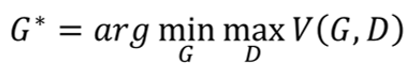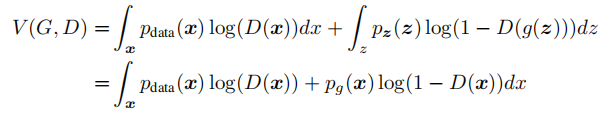=》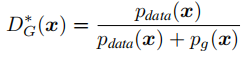固定G，update D with gradient ascent（最优D：最大D）update：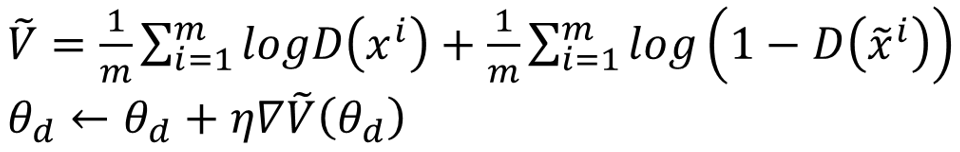通过JS divergence 度量pdata 和pG 之间相似度（距离）
寻找minimize JS
训练Generator
update G: gradiant descent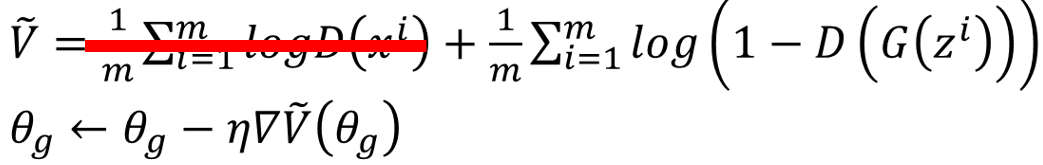Problem
Don’t update G too much
JS divergence is log2 if two distributions do not overlap
add noise to the inputs of discriminator (decay over time)
mode collapse
Least Square GAN (LSGAN): Linear
replace sigmoid with linear (replace classification with regression)
WGAN: Earth Mover’s Distance
展开全文• 原始生成对抗网络源码，通过利用现有的手写数字的数据集MNIST，我通过GAN生成一些能够以假乱真的手写字图片
• GAN图像优化 GAN图像优化 GAN图像优化 GAN图像优化 GAN图像优化 GAN图像优化 GAN图像优化 GAN图像优化 GAN图像优化
• 我们为CA-GAN和SCA-GAN提供PyTorch实施。 论文“通过合成辅助GAN实现逼真的面部照片素描合成” [ 发电机架构 样品结果 左：草图合成； 右：照片合成 （a）输入图像，（b）cGAN，（c）CA-GAN，（d）SCA-GAN 先决...
• 将VAE和GAN相结合，通过利用GAN鉴别器中的学习特征表示作为VAE重建目标的基础，提高生成效果
• GAN教程 GAN论文简短回顾练习GAN-GAN_for_MNIST_Tutorial.ipynb
• tensorflow搭建简单GAN网络，生成手写数字图片
• 应用Keras实现GAN网络的实现，用于学习基本的知识，新手练习使用
• GAN protocol raylieh channel
• GAN for toonsquare GAN着色 要求 张量流2.2.0 python 3.6.10Python
• ## 生成对抗网络——GAN（一）

万次阅读 多人点赞 2018-07-30 23:38:08
由此可见，GAN在视觉领域的未来多年内，将是一片沃土(CVer们是时候入门GAN了)。而发现这片矿源的就是GAN之父，Goodfellow大神。 ~~~ 生成对抗网络GAN，是当今的一大热门研究方向。在2014年，被Goodfellow大神提...
Generative adversarial network
据有关媒体统计：CVPR2018的论文里，有三分之一的论文与GAN有关 由此可见，GAN在视觉领域的未来多年内，将是一片沃土(CVer们是时候入门GAN了)。而发现这片矿源的就是GAN之父，Goodfellow大神。 文末有基于keras的GAN代码，有助于理解GAN的原理

生成对抗网络GAN，是当今的一大热门研究方向。在2014年，被Goodfellow大神提出来，当时的G神还只是蒙特利尔大学的博士生而已。 GAN之父的主页： http://www.iangoodfellow.com/
GAN的论文首次出现在NIPS2014上，论文地址如下： https://arxiv.org/pdf/1406.2661.pdf

入坑GAN，首先需要理由，GAN能做什么，为什么要学GAN。 GAN的初衷就是生成不存在于真实世界的数据，类似于使得 AI具有创造力或者想象力。应用场景如下：
AI作家，AI画家等需要创造力的AI体；将模糊图变清晰(去雨，去雾，去抖动，去马赛克等)，这需要AI具有所谓的“想象力”，能脑补情节；进行数据增强，根据已有数据生成更多新数据供以feed，可以减缓模型过拟合现象。 以上的场景都可以找到相应的paper。而且GAN的用处也远不止此，期待我们继续挖掘，是发论文的好方向哦

GAN的原理介绍
这里介绍的是原生的GAN算法，虽然有一些不足，但提供了一种生成对抗性的新思路。放心，我这篇博文不会堆一大堆公式，只会提供一种理解思路。
理解GAN的两大护法G和D
G是generator，生成器: 负责凭空捏造数据出来
D是discriminator，判别器: 负责判断数据是不是真数据
这样可以简单的看作是两个网络的博弈过程。在最原始的GAN论文里面，G和D都是两个多层感知机网络。首先，注意一点，GAN操作的数据不一定非得是图像数据，不过为了更方便解释，我在这里用图像数据为例解释以下GAN：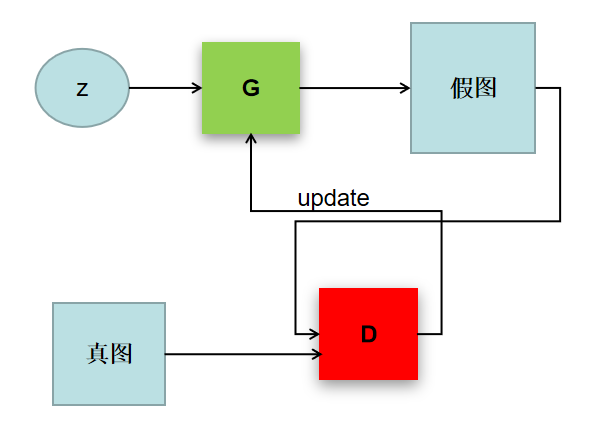稍微解释以下上图，z是随机噪声(就是随机生成的一些数，也就是GAN生成图像的源头)。D通过真图和假图的数据(相当于天然label)，进行一个二分类神经网络训练(想各位必再熟悉不过了)。G根据一串随机数就可以捏造一个“假图像”出来，用这些假图去欺骗D，D负责辨别这是真图还是假图，会给出一个score。比如，G生成了一张图，在D这里得分很高，那证明G是很成功的；如果D能有效区分真假图，则G的效果还不太好，需要调整参数。GAN就是这么一个博弈的过程。

那么，GAN是怎么训练呢？ 根据GAN的训练算法，我画一张图：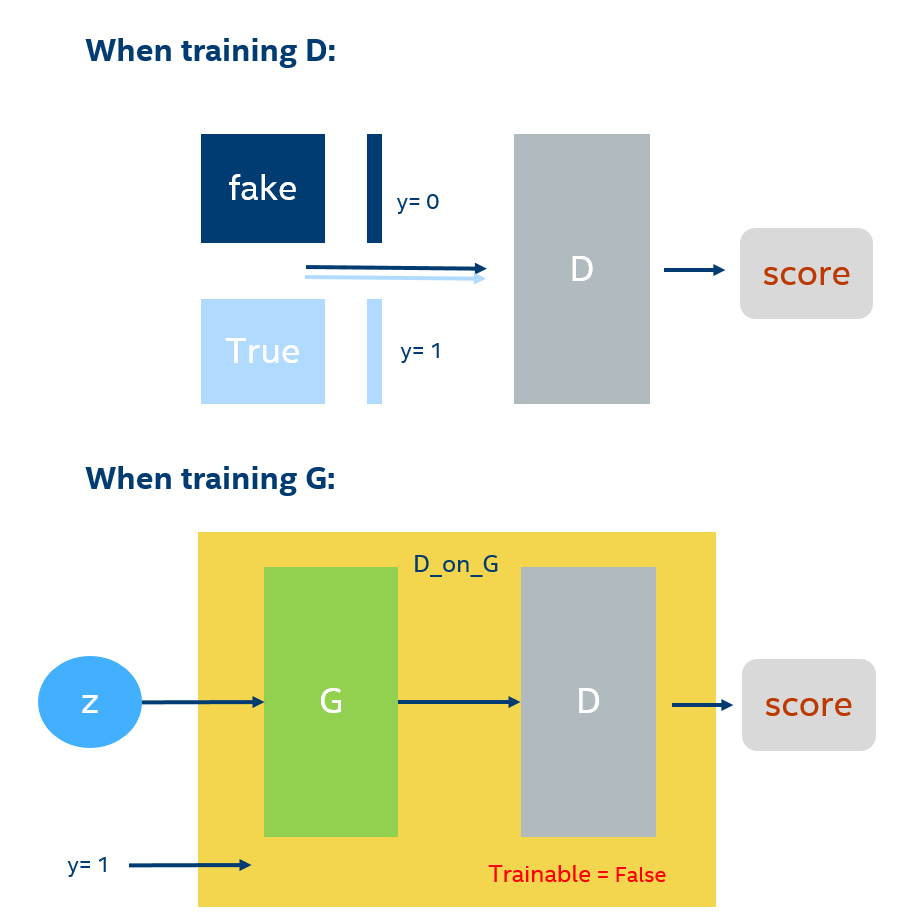GAN的训练在同一轮梯度反传的过程中可以细分为2步，先训练D在训练G；注意不是等所有的D训练好以后，才开始训练G，因为D的训练也需要上一轮梯度反传中G的输出值作为输入。  当训练D的时候，上一轮G产生的图片，和真实图片，直接拼接在一起，作为x。然后根据，按顺序摆放0和1，假图对应0，真图对应1。然后就可以通过，x输入生成一个score（从0到1之间的数），通过score和y组成的损失函数，就可以进行梯度反传了。（我在图片上举的例子是batch = 1，len(y)=2*batch，训练时通常可以取较大的batch）
当训练G的时候， 需要把G和D当作一个整体，我在这里取名叫做’D_on_G’。这个整体(下面简称DG系统)的输出仍然是score。输入一组随机向量，就可以在G生成一张图，通过D对生成的这张图进行打分，这就是DG系统的前向过程。score=1就是DG系统需要优化的目标，score和y=1之间的差异可以组成损失函数，然后可以反向传播梯度。注意，这里的D的参数是不可训练的。这样就能保证G的训练是符合D的打分标准的。这就好比：如果你参加考试，你别指望能改变老师的评分标准

需要注意的是，整个GAN的整个过程都是无监督的(后面会有监督性GAN比如cGAN)，怎么理解这里的无监督呢？ 这里，给的真图是没有经过人工标注的，你只知道这是真实的图片，比如全是人脸，而系统里的D并不知道来的图片是什么玩意儿，它只需要分辨真假。G也不知道自己生成的是什么玩意儿，反正就是学真图片的样子骗D。
正由于GAN的无监督，在生成过程中，G就会按照自己的意思天马行空生成一些“诡异”的图片，可怕的是D还能给一个很高的分数。比如，生成人脸极度扭曲的图片。这就是无监督目的性不强所导致的，所以在同年的NIPS大会上，有一篇论文conditional GAN就加入了监督性进去，将可控性增强，表现效果也好很多。

from __future__ import print_function, division

from keras.datasets import mnist
from keras.layers import Input, Dense, Reshape, Flatten, Dropout
from keras.layers import BatchNormalization, Activation, ZeroPadding2D
from keras.layers.convolutional import UpSampling2D, Conv2D
from keras.models import Sequential, Model

import matplotlib.pyplot as plt

import sys

import numpy as np

class GAN():
def __init__(self):
self.img_rows = 28
self.img_cols = 28
self.channels = 1
self.img_shape = (self.img_rows, self.img_cols, self.channels)
self.latent_dim = 100

# Build and compile the discriminator
self.discriminator = self.build_discriminator()
self.discriminator.compile(loss='binary_crossentropy',
optimizer=optimizer,
metrics=['accuracy'])

# Build the generator
self.generator = self.build_generator()

# The generator takes noise as input and generates imgs
z = Input(shape=(self.latent_dim,))
img = self.generator(z)

# For the combined model we will only train the generator
self.discriminator.trainable = False

# The discriminator takes generated images as input and determines validity
validity = self.discriminator(img)

# The combined model  (stacked generator and discriminator)
# Trains the generator to fool the discriminator
self.combined = Model(z, validity)
self.combined.compile(loss='binary_crossentropy', optimizer=optimizer)

def build_generator(self):

model = Sequential()

model.summary()

noise = Input(shape=(self.latent_dim,))
img = model(noise)

return Model(noise, img)

def build_discriminator(self):

model = Sequential()

model.summary()

img = Input(shape=self.img_shape)
validity = model(img)

return Model(img, validity)

def train(self, epochs, batch_size=128, sample_interval=50):

(X_train, _), (_, _) = mnist.load_data()

# Rescale -1 to 1
X_train = X_train / 127.5 - 1.
X_train = np.expand_dims(X_train, axis=3)

valid = np.ones((batch_size, 1))
fake = np.zeros((batch_size, 1))

for epoch in range(epochs):

# ---------------------
#  Train Discriminator
# ---------------------

# Select a random batch of images
idx = np.random.randint(0, X_train.shape, batch_size)
imgs = X_train[idx]

noise = np.random.normal(0, 1, (batch_size, self.latent_dim))

# Generate a batch of new images
gen_imgs = self.generator.predict(noise)

# Train the discriminator
d_loss_real = self.discriminator.train_on_batch(imgs, valid)
d_loss_fake = self.discriminator.train_on_batch(gen_imgs, fake)
d_loss = 0.5 * np.add(d_loss_real, d_loss_fake)

# ---------------------
#  Train Generator
# ---------------------

noise = np.random.normal(0, 1, (batch_size, self.latent_dim))

# Train the generator (to have the discriminator label samples as valid)
g_loss = self.combined.train_on_batch(noise, valid)

# Plot the progress
print ("%d [D loss: %f, acc.: %.2f%%] [G loss: %f]" % (epoch, d_loss, 100*d_loss, g_loss))

# If at save interval => save generated image samples
if epoch % sample_interval == 0:
self.sample_images(epoch)

def sample_images(self, epoch):
r, c = 5, 5
noise = np.random.normal(0, 1, (r * c, self.latent_dim))
gen_imgs = self.generator.predict(noise)

# Rescale images 0 - 1
gen_imgs = 0.5 * gen_imgs + 0.5

fig, axs = plt.subplots(r, c)
cnt = 0
for i in range(r):
for j in range(c):
axs[i,j].imshow(gen_imgs[cnt, :,:,0], cmap='gray')
axs[i,j].axis('off')
cnt += 1
fig.savefig("images/%d.png" % epoch)
plt.close()

if __name__ == '__main__':
gan = GAN()
gan.train(epochs=30000, batch_size=32, sample_interval=200)

展开全文• 围栏GAN：寻求更好的异常检测 这是本文的正式实施：围栏GAN：迈向更好的异常检测 。 先决条件 Linux操作系统 的Python 3 CUDA Tensorflow版本1.12（已在此版本上测试） 安装 克隆存储库 git clone ...
• 使用KERAS作为框架搭建了一个·GAN进行脊柱分割
• ## 详解GAN代码之逐行解析GAN代码

万次阅读 多人点赞 2018-04-21 21:28:53
训练数据集：手写数字识别下载链接：https://pan.baidu.com/s/1d9jX5xLHd1x3DFChVCe3LQ 密码：ws28 在本篇博客中，笔者将逐行解析一下NIPS 2014的Generative Adversarial Networks(生成对抗网络，简称GAN)代码，该...
训练数据集：手写数字识别
下载链接：https://pan.baidu.com/s/1d9jX5xLHd1x3DFChVCe3LQ 密码：ws28
在正式开始之前，笔者想说的是，如果要使得本篇博客对各位读者朋友的学习有帮助，请各位读者朋友们先熟悉生成对抗网络的基本原理。由于对于生成对抗网络的原理详解网络上的资源比较多，在本篇博客中笔者就不再对生成对抗网络的原理进行解释，而是给大家推荐一些对生成对抗网络原理进行了解的链接：
1. 直接进行论文阅读：https://arxiv.org/abs/1406.2661
2. 一篇通俗易懂，形象的GAN原理解释：一文看懂生成式对抗网络GANs：介绍指南及前景展望
3. 一篇比较详细的CSDN博文：生成式对抗网络GAN研究进展（二）——原始GAN
4. 知乎专栏上的文章：GAN原理学习笔记
如果对生成对抗网络原理已经熟稔的读者朋友，请自动忽略以上链接。并且，笔者以下放出的代码注释是参考了github上面的代码，链接https://github.com/wiseodd/generative-models
在这里笔者也想衷心感谢一下这位wiseodd大神，在他的generative-models下面的关于生成模型的代码非常全面，本文解析的代码路径是该工程下面的GAN/vanilla_gan/gan_tensorflow.py文件。笔者沿用了作者的代码，只是增加了模型保存与summary记录的少量代码，下面放出代码及注释：
import tensorflow as tf #导入tensorflow
from tensorflow.examples.tutorials.mnist import input_data #导入手写数字数据集
import numpy as np #导入numpy
import matplotlib.pyplot as plt #plt是绘图工具，在训练过程中用于输出可视化结果
import matplotlib.gridspec as gridspec #gridspec是图片排列工具，在训练过程中用于输出可视化结果
import os #导入os

def save(saver, sess, logdir, step): #保存模型的save函数
model_name = 'model' #模型名前缀
checkpoint_path = os.path.join(logdir, model_name) #保存路径
saver.save(sess, checkpoint_path, global_step=step) #保存模型
print('The checkpoint has been created.')

def xavier_init(size): #初始化参数时使用的xavier_init函数
in_dim = size
xavier_stddev = 1. / tf.sqrt(in_dim / 2.) #初始化标准差
return tf.random_normal(shape=size, stddev=xavier_stddev) #返回初始化的结果

X = tf.placeholder(tf.float32, shape=[None, 784]) #X表示真的样本(即真实的手写数字)

D_W1 = tf.Variable(xavier_init([784, 128])) #表示使用xavier方式初始化的判别器的D_W1参数，是一个784行128列的矩阵
D_b1 = tf.Variable(tf.zeros(shape=)) #表示全零方式初始化的判别器的D_1参数，是一个长度为128的向量

D_W2 = tf.Variable(xavier_init([128, 1])) #表示使用xavier方式初始化的判别器的D_W2参数，是一个128行1列的矩阵
D_b2 = tf.Variable(tf.zeros(shape=)) ##表示全零方式初始化的判别器的D_1参数，是一个长度为1的向量

theta_D = [D_W1, D_W2, D_b1, D_b2] #theta_D表示判别器的可训练参数集合

Z = tf.placeholder(tf.float32, shape=[None, 100]) #Z表示生成器的输入(在这里是噪声)，是一个N列100行的矩阵

G_W1 = tf.Variable(xavier_init([100, 128])) #表示使用xavier方式初始化的生成器的G_W1参数，是一个100行128列的矩阵
G_b1 = tf.Variable(tf.zeros(shape=)) #表示全零方式初始化的生成器的G_b1参数，是一个长度为128的向量

G_W2 = tf.Variable(xavier_init([128, 784])) #表示使用xavier方式初始化的生成器的G_W2参数，是一个128行784列的矩阵
G_b2 = tf.Variable(tf.zeros(shape=)) #表示全零方式初始化的生成器的G_b2参数，是一个长度为784的向量

theta_G = [G_W1, G_W2, G_b1, G_b2] #theta_G表示生成器的可训练参数集合

def sample_Z(m, n): #生成维度为[m, n]的随机噪声作为生成器G的输入
return np.random.uniform(-1., 1., size=[m, n])

def generator(z): #生成器，z的维度为[N, 100]
G_h1 = tf.nn.relu(tf.matmul(z, G_W1) + G_b1) #输入的随机噪声乘以G_W1矩阵加上偏置G_b1，G_h1维度为[N, 128]
G_log_prob = tf.matmul(G_h1, G_W2) + G_b2 #G_h1乘以G_W2矩阵加上偏置G_b2，G_log_prob维度为[N, 784]
G_prob = tf.nn.sigmoid(G_log_prob) #G_log_prob经过一个sigmoid函数，G_prob维度为[N, 784]

return G_prob #返回G_prob

def discriminator(x): #判别器，x的维度为[N, 784]
D_h1 = tf.nn.relu(tf.matmul(x, D_W1) + D_b1) #输入乘以D_W1矩阵加上偏置D_b1，D_h1维度为[N, 128]
D_logit = tf.matmul(D_h1, D_W2) + D_b2 #D_h1乘以D_W2矩阵加上偏置D_b2，D_logit维度为[N, 1]
D_prob = tf.nn.sigmoid(D_logit) #D_logit经过一个sigmoid函数，D_prob维度为[N, 1]

return D_prob, D_logit #返回D_prob, D_logit

def plot(samples): #保存图片时使用的plot函数
fig = plt.figure(figsize=(4, 4)) #初始化一个4行4列包含16张子图像的图片
gs = gridspec.GridSpec(4, 4) #调整子图的位置
gs.update(wspace=0.05, hspace=0.05) #置子图间的间距

for i, sample in enumerate(samples): #依次将16张子图填充进需要保存的图像
ax = plt.subplot(gs[i])
plt.axis('off')
ax.set_xticklabels([])
ax.set_yticklabels([])
ax.set_aspect('equal')
plt.imshow(sample.reshape(28, 28), cmap='Greys_r')

return fig

G_sample = generator(Z) #取得生成器的生成结果
D_real, D_logit_real = discriminator(X) #取得判别器判别的真实手写数字的结果
D_fake, D_logit_fake = discriminator(G_sample) #取得判别器判别的生成的手写数字的结果

D_loss_real = tf.reduce_mean(tf.nn.sigmoid_cross_entropy_with_logits(logits=D_logit_real, labels=tf.ones_like(D_logit_real))) #对判别器对真实样本的判别结果计算误差(将结果与1比较)
D_loss_fake = tf.reduce_mean(tf.nn.sigmoid_cross_entropy_with_logits(logits=D_logit_fake, labels=tf.zeros_like(D_logit_fake))) #对判别器对虚假样本(即生成器生成的手写数字)的判别结果计算误差(将结果与0比较)
D_loss = D_loss_real + D_loss_fake #判别器的误差
G_loss = tf.reduce_mean(tf.nn.sigmoid_cross_entropy_with_logits(logits=D_logit_fake, labels=tf.ones_like(D_logit_fake))) #生成器的误差(将判别器返回的对虚假样本的判别结果与1比较)

dreal_loss_sum = tf.summary.scalar("dreal_loss", D_loss_real) #记录判别器判别真实样本的误差
dfake_loss_sum = tf.summary.scalar("dfake_loss", D_loss_fake) #记录判别器判别虚假样本的误差
d_loss_sum = tf.summary.scalar("d_loss", D_loss) #记录判别器的误差
g_loss_sum = tf.summary.scalar("g_loss", G_loss) #记录生成器的误差

summary_writer = tf.summary.FileWriter('snapshots/', graph=tf.get_default_graph()) #日志记录器

mb_size = 128 #训练的batch_size
Z_dim = 100 #生成器输入的随机噪声的列的维度

sess = tf.Session() #会话层
sess.run(tf.global_variables_initializer()) #初始化所有可训练参数

if not os.path.exists('out/'): #初始化训练过程中的可视化结果的输出文件夹
os.makedirs('out/')

if not os.path.exists('snapshots/'): #初始化训练过程中的模型保存文件夹
os.makedirs('snapshots/')

saver = tf.train.Saver(var_list=tf.global_variables(), max_to_keep=50) #模型的保存器

i = 0 #训练过程中保存的可视化结果的索引

for it in range(1000000): #训练100万次
if it % 1000 == 0: #每训练1000次就保存一下结果
samples = sess.run(G_sample, feed_dict={Z: sample_Z(16, Z_dim)})

fig = plot(samples) #通过plot函数生成可视化结果
plt.savefig('out/{}.png'.format(str(i).zfill(3)), bbox_inches='tight') #保存可视化结果
i += 1
plt.close(fig)

X_mb, _ = mnist.train.next_batch(mb_size) #得到训练一个batch所需的真实手写数字(作为判别器的输入)

#下面是得到训练一次的结果，通过sess来run出来
_, D_loss_curr, dreal_loss_sum_value, dfake_loss_sum_value, d_loss_sum_value = sess.run([D_solver, D_loss, dreal_loss_sum, dfake_loss_sum, d_loss_sum], feed_dict={X: X_mb, Z: sample_Z(mb_size, Z_dim)})
_, G_loss_curr, g_loss_sum_value = sess.run([G_solver, G_loss, g_loss_sum], feed_dict={Z: sample_Z(mb_size, Z_dim)})

if it%100 ==0: #每过100次记录一下日志，可以通过tensorboard查看

if it % 1000 == 0: #每训练1000次输出一下结果
save(saver, sess, 'snapshots/', it)
print('Iter: {}'.format(it))
print('D loss: {:.4}'. format(D_loss_curr))
print('G_loss: {:.4}'.format(G_loss_curr))
print()
在上面的代码中，笔者添加了各种summary保存了训练中的误差，结果如下所示。
判别器判别真实样本的误差变化：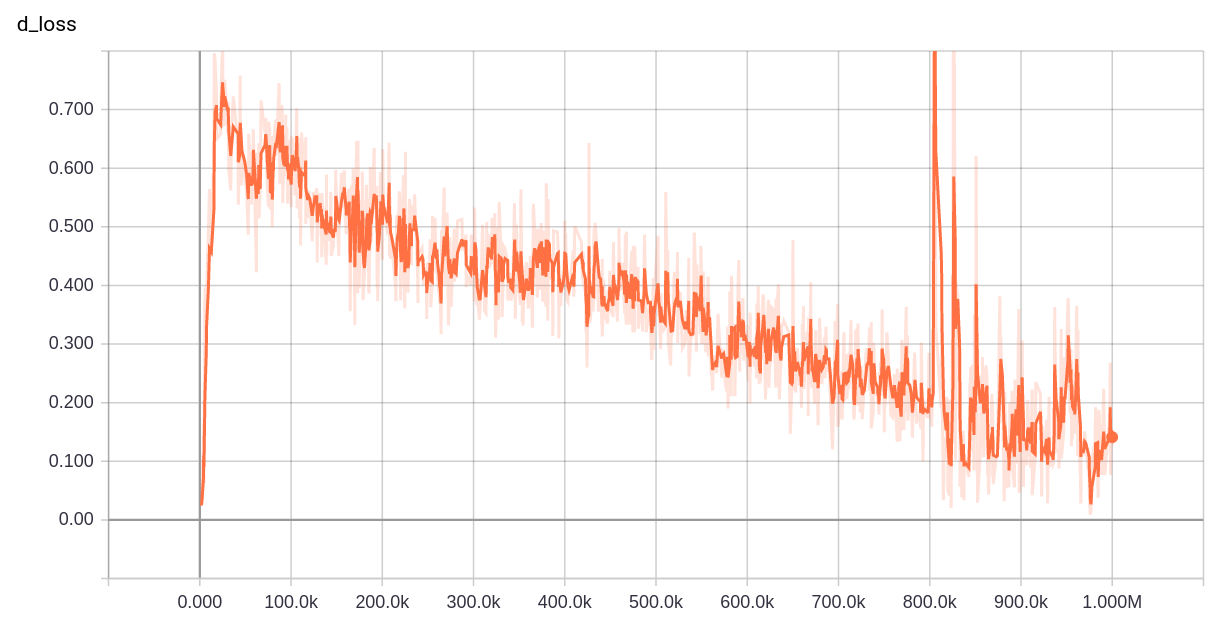判别器判别虚假样本(即生成器G生成的手写数字)的误差变化：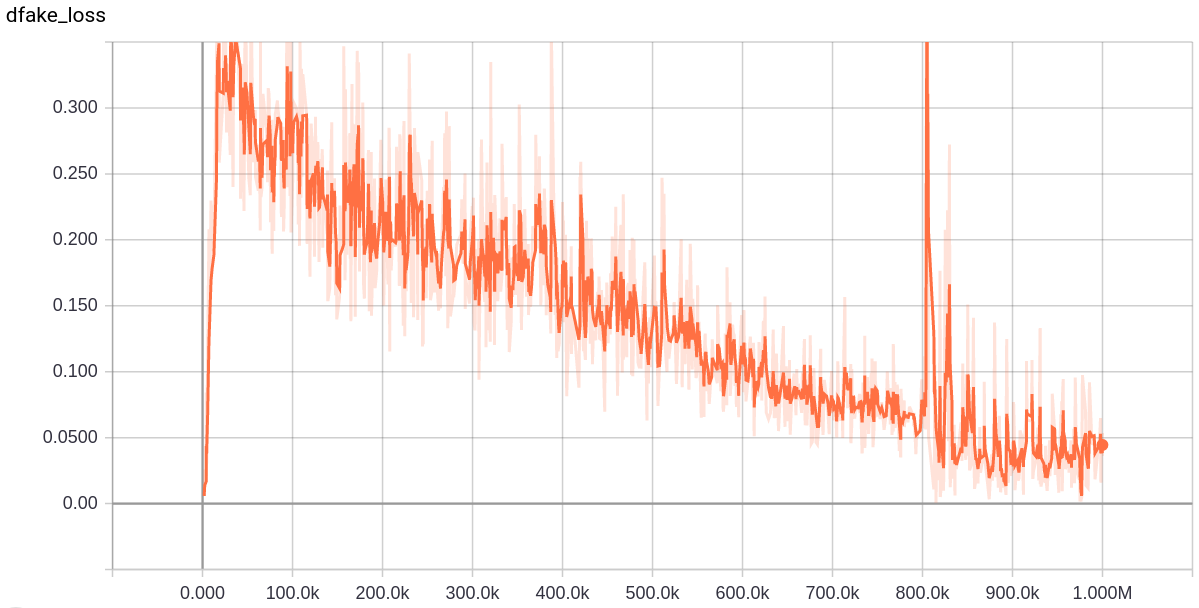判别器的误差变化(上面两者之和)：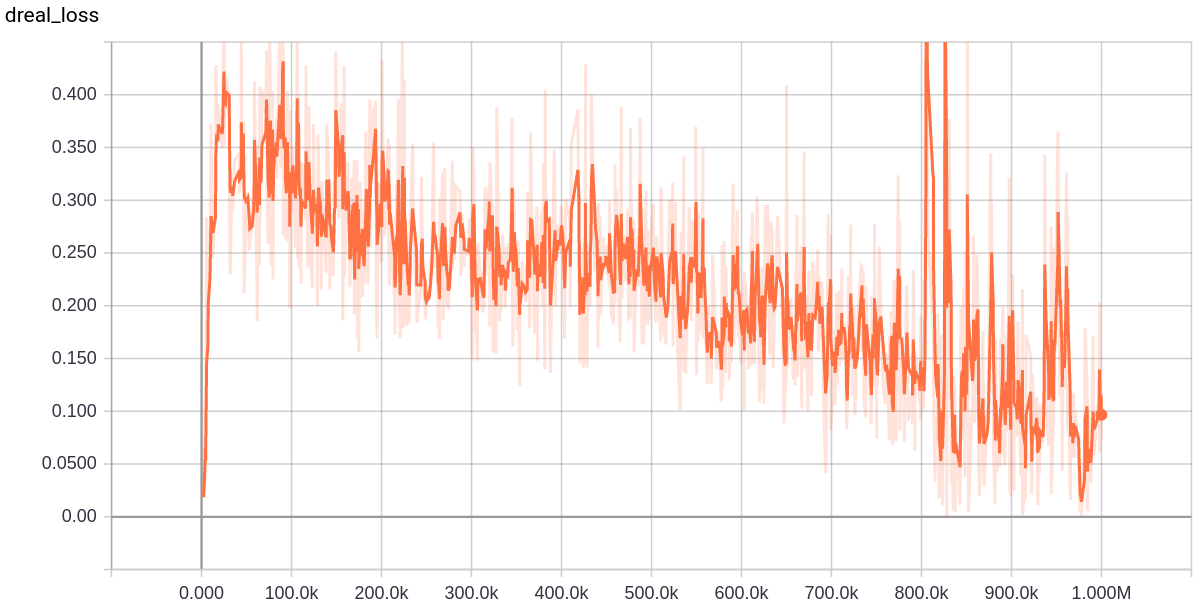生成器的误差变化：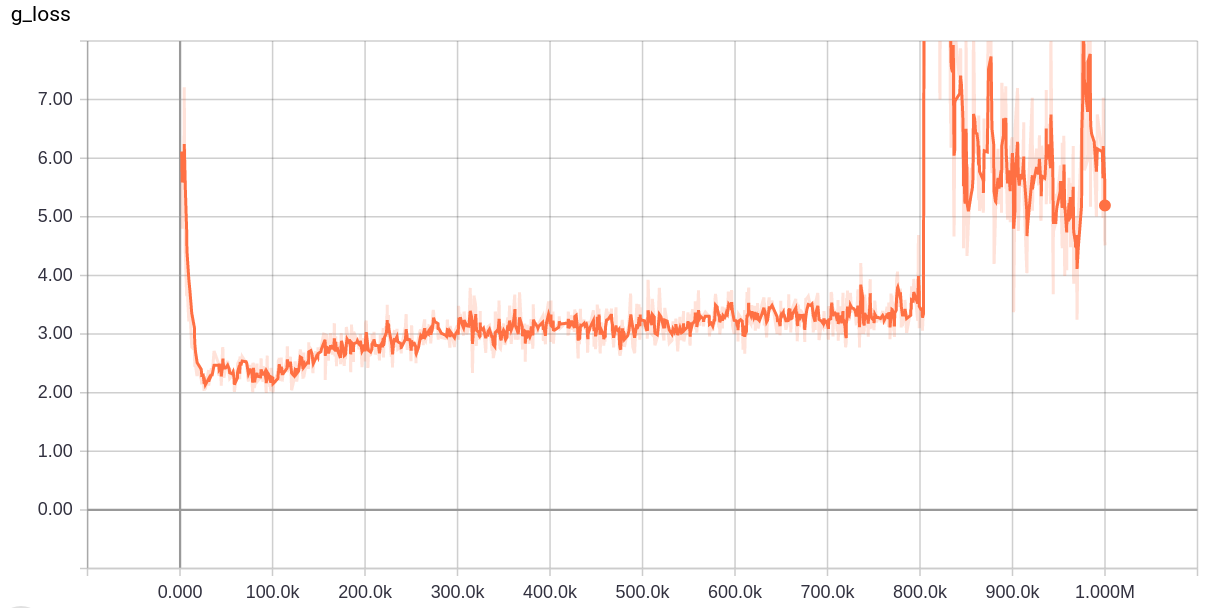下面是训练过程中输出的可视化结果，笔者选择了一些，大家可以看到，生成器输出结果最开始非常糟糕，但是随着训练的进行到训练中期输出效果越来越好：
训练2k次的输出：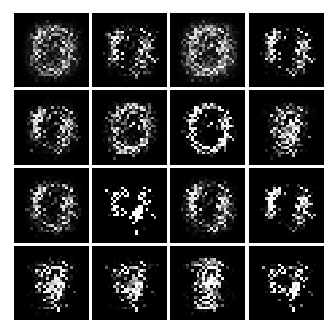训练6k次的输出：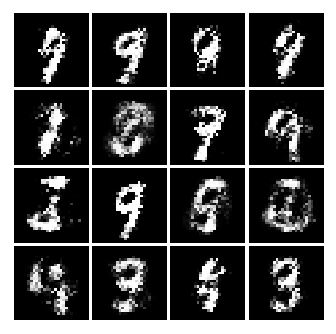训练4.2w次的输出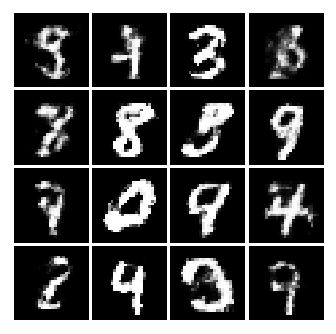训练14.4w次的输出：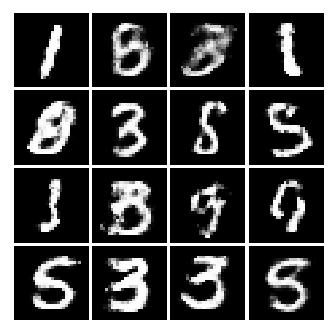训练24.4w次的输出：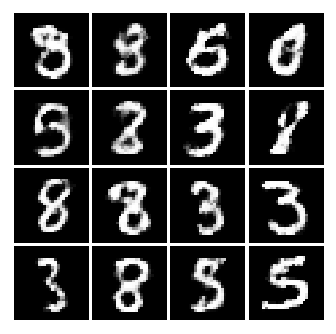训练31.6w次的输出：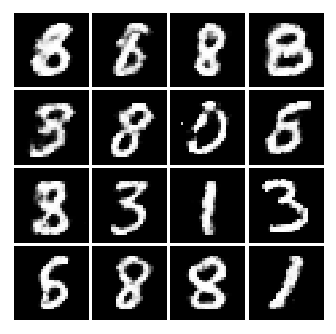在训练的后期(训练80w次之后)，大家从生成器的误差曲线可以看出，生成器的误差陡增，生成效果也相应变差了(如下图所示)，这是生成器与判别器失衡的结果。
训练85.7w次的输出：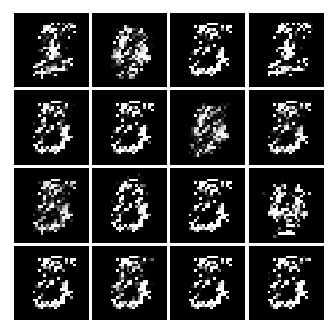训练93.6w次的输出：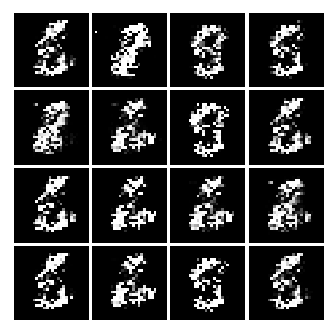训练97.2w次的输出：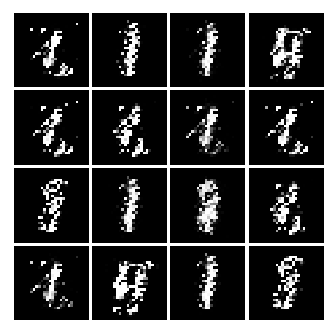到这里，生成对抗网络的代码讲解就接近尾声了，衷心希望笔者的本篇博客对大家有帮助！
欢迎阅读笔者后续博客，各位读者朋友的支持与鼓励是我最大的动力！

written by jiong
日出入安穷？时世不与人同。
故春非我春，夏非我夏，秋非我秋，
冬，亦非我冬。
展开全文生成对抗网络 tensorflow 手写数字
• TensorFlow-Conditional-GAN （Conditional-GAN）条件生成对抗网络 可以使用Vanilla GAN模型创建所需目标图像的模型 有条件的GAN论文： : 有条件的GAN.py * loss function는 ...
• GAN-教程 PyTorch 中的 GAN 和 DCGAN 教程 GAN.ipynb 使用线性层在 MNIST 上训练 GAN。 论文： : GAN_generation_images 它是一个包含学习 GAN 时创建的生成结果的文件夹。 还包括通过连接图像创建的 .gif。 ...
• 利用TensorFlow框架做的简单的GAN网络运行jupyter notebook
• GaN∶Eu 3+作为红光发射材料,在GaN基单片集成全色显示器件应用方面具有很大的潜力。目前的研究重点是如何进一步调控和优化GaN∶Eu 3+材料的发光特性,促使其迈向实用阶段。本文主要从生长调控,Mg 2+、Zn 2+、Si 4+...
• ## GaN/Al

2021-02-11 06:08:43
在Al2O3(0001)衬底上用MOCVD(金属有机物气相沉积)方法进行了GaN的外延生长,通过Ｘ射线衍射(同步辐射源)研究了GaN和Al2O3(0001)的匹配关系。结果表明,经充分氮化的衬底上,GaN以单一的匹配方式沿［0001］方向生长;在Al...
• ## 简单理解与实验生成对抗网络GAN

万次阅读 多人点赞 2017-05-26 21:31:49
之前GAN网络是近两年深度学习领域的新秀，火的不行，本文旨在浅显理解传统GAN，分享学习心得。现有GAN网络大多数代码实现使用python、torch等语言，这里，后面用matlab搭建一个简单的GAN网络，便于理解GAN原理。GAN...
之前
GAN网络是近两年深度学习领域的新秀，火的不行，本文旨在浅显理解传统GAN，分享学习心得。现有GAN网络大多数代码实现使用python、torch等语言，这里，后面用matlab搭建一个简单的GAN网络，便于理解GAN原理。
分享一个目前各类GAN的一个论文整理集合再分享一个目前各类GAN的一个代码整理集合
开始
我们知道GAN的思想是是一种二人零和博弈思想（two-player game），博弈双方的利益之和是一个常数，比如两个人掰手腕，假设总的空间是一定的，你的力气大一点，那你就得到的空间多一点，相应的我的空间就少一点，相反我力气大我就得到的多一点，但有一点是确定的就是，我两的总空间是一定的，这就是二人博弈，但是呢总利益是一定的。
引申到GAN里面就是可以看成，GAN中有两个这样的博弈者，一个人名字是生成模型（G），另一个人名字是判别模型（D）。他们各自有各自的功能。
相同点是：
这两个模型都可以看成是一个黑匣子，接受输入然后有一个输出，类似一个函数，一个输入输出映射。
不同点是：
生成模型功能：比作是一个样本生成器，输入一个噪声/样本，然后把它包装成一个逼真的样本，也就是输出。判别模型：比作一个二分类器（如同0-1分类器），来判断输入的样本是真是假。（就是输出值大于0.5还是小于0.5）;
直接上一张个人觉得解释的好的图说明：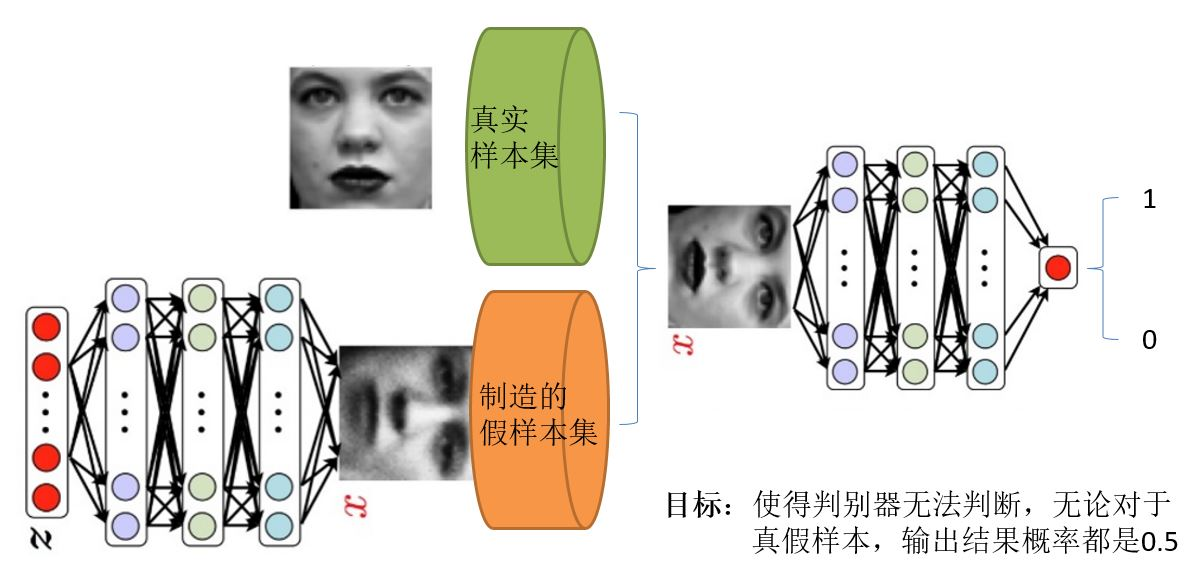在之前，我们首先明白在使用GAN的时候的2个问题
我们有什么？  比如上面的这个图，我们有的只是真实采集而来的人脸样本数据集，仅此而已，而且很关键的一点是我们连人脸数据集的类标签都没有，也就是我们不知道那个人脸对应的是谁。我们要得到什么  至于要得到什么，不同的任务得到的东西不一样，我们只说最原始的GAN目的，那就是我们想通过输入一个噪声，模拟得到一个人脸图像，这个图像可以非常逼真以至于以假乱真。
好了再来理解下GAN的两个模型要做什么。首先判别模型，就是图中右半部分的网络，直观来看就是一个简单的神经网络结构，输入就是一副图像，输出就是一个概率值，用于判断真假使用（概率值大于0.5那就是真，小于0.5那就是假），真假也不过是人们定义的概率而已。其次是生成模型，生成模型要做什么呢，同样也可以看成是一个神经网络模型，输入是一组随机数Z，输出是一个图像，不再是一个数值而已。从图中可以看到，会存在两个数据集，一个是真实数据集，这好说，另一个是假的数据集，那这个数据集就是有生成网络造出来的数据集。好了根据这个图我们再来理解一下GAN的目标是要干什么：
判别网络的目的：就是能判别出来属于的一张图它是来自真实样本集还是假样本集。假如输入的是真样本，网络输出就接近1，输入的是假样本，网络输出接近0，那么很完美，达到了很好判别的目的。生成网络的目的：生成网络是造样本的，它的目的就是使得自己造样本的能力尽可能强，强到什么程度呢，你判别网络没法判断我是真样本还是假样本。
有了这个理解我们再来看看为什么叫做对抗网络了。判别网络说，我很强，来一个样本我就知道它是来自真样本集还是假样本集。生成网络就不服了，说我也很强，我生成一个假样本，虽然我生成网络知道是假的，但是你判别网络不知道呀，我包装的非常逼真，以至于判别网络无法判断真假，那么用输出数值来解释就是，生成网络生成的假样本进去了判别网络以后，判别网络给出的结果是一个接近0.5的值，极限情况就是0.5，也就是说判别不出来了，这就是纳什平衡了。
由这个分析可以发现，生成网络与判别网络的目的正好是相反的，一个说我能判别的好，一个说我让你判别不好。所以叫做对抗，叫做博弈。那么最后的结果到底是谁赢呢？这就要归结到设计者，也就是我们希望谁赢了。作为设计者的我们，我们的目的是要得到以假乱真的样本，那么很自然的我们希望生成样本赢了，也就是希望生成样本很真，判别网络能力不足以区分真假样本位置。
再理解
知道了GAN大概的目的与设计思路，那么一个很自然的问题来了就是我们该如何用数学方法解决这么一个对抗问题。这就涉及到如何训练这样一个生成对抗网络模型了，还是先上一个图，用图来解释最直接：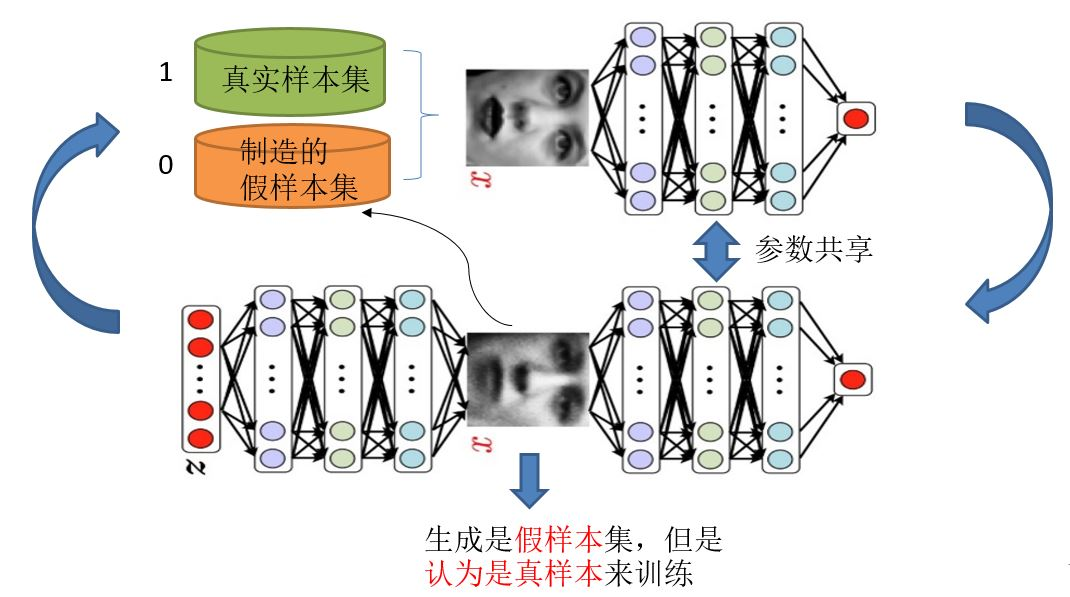需要注意的是生成模型与对抗模型可以说是完全独立的两个模型，好比就是完全独立的两个神经网络模型，他们之间没有什么联系。
好了那么训练这样的两个模型的大方法就是：单独交替迭代训练。
什么意思？因为是2个网络，不好一起训练，所以才去交替迭代训练，我们一一来看。  假设现在生成网络模型已经有了（当然可能不是最好的生成网络），那么给一堆随机数组，就会得到一堆假的样本集（因为不是最终的生成模型，那么现在生成网络可能就处于劣势，导致生成的样本就不咋地，可能很容易就被判别网络判别出来了说这货是假冒的），但是先不管这个，假设我们现在有了这样的假样本集，真样本集一直都有，现在我们人为的定义真假样本集的标签，因为我们希望真样本集的输出尽可能为1，假样本集为0，很明显这里我们就已经默认真样本集所有的类标签都为1，而假样本集的所有类标签都为0. 有人会说，在真样本集里面的人脸中，可能张三人脸和李四人脸不一样呀，对于这个问题我们需要理解的是，我们现在的任务是什么，我们是想分样本真假，而不是分真样本中那个是张三label、那个是李四label。况且我们也知道，原始真样本的label我们是不知道的。回过头来，我们现在有了真样本集以及它们的label（都是1）、假样本集以及它们的label（都是0），这样单就判别网络来说，此时问题就变成了一个再简单不过的有监督的二分类问题了，直接送到神经网络模型中训练就完事了。假设训练完了，下面我们来看生成网络。
对于生成网络，想想我们的目的，是生成尽可能逼真的样本。那么原始的生成网络生成的样本你怎么知道它真不真呢？就是送到判别网络中，所以在训练生成网络的时候，我们需要联合判别网络一起才能达到训练的目的。什么意思？就是如果我们单单只用生成网络，那么想想我们怎么去训练？误差来源在哪里？细想一下没有，但是如果我们把刚才的判别网络串接在生成网络的后面，这样我们就知道真假了，也就有了误差了。所以对于生成网络的训练其实是对生成-判别网络串接的训练，就像图中显示的那样。好了那么现在来分析一下样本，原始的噪声数组Z我们有，也就是生成了假样本我们有，此时很关键的一点来了，我们要把这些假样本的标签都设置为1，也就是认为这些假样本在生成网络训练的时候是真样本。那么为什么要这样呢？我们想想，是不是这样才能起到迷惑判别器的目的，也才能使得生成的假样本逐渐逼近为正样本。好了，重新顺一下思路，现在对于生成网络的训练，我们有了样本集（只有假样本集，没有真样本集），有了对应的label（全为1），是不是就可以训练了？有人会问，这样只有一类样本，训练啥呀？谁说一类样本就不能训练了？只要有误差就行。还有人说，你这样一训练，判别网络的网络参数不是也跟着变吗？没错，这很关键，所以在训练这个串接的网络的时候，一个很重要的操作就是不要判别网络的参数发生变化，也就是不让它参数发生更新，只是把误差一直传，传到生成网络那块后更新生成网络的参数。这样就完成了生成网络的训练了。
在完成生成网络训练好，那么我们是不是可以根据目前新的生成网络再对先前的那些噪声Z生成新的假样本了，没错，并且训练后的假样本应该是更真了才对。然后又有了新的真假样本集（其实是新的假样本集），这样又可以重复上述过程了。我们把这个过程称作为单独交替训练。我们可以实现定义一个迭代次数，交替迭代到一定次数后停止即可。这个时候我们再去看一看噪声Z生成的假样本会发现，原来它已经很真了。
看完了这个过程是不是感觉GAN的设计真的很巧妙，个人觉得最值得称赞的地方可能在于这种假样本在训练过程中的真假变换，这也是博弈得以进行的关键之处。
进一步
文字的描述相信已经让大多数的人知道了这个过程，下面我们来看看原文中几个重要的数学公式描述，首先我们直接上原始论文中的目标公式吧：

minGmaxDV(D,G)=Ex∼pdata(x)[log(D(x))]+Ez∼pz(z)[log(1−D(G(z)))]

\min \limits_G \max \limits_D V(D,G)=E_{x \sim p_{data}(x)}[log(D(x))]+E_{z \sim p_{z}(z)}[log(1-D(G(z)))]

上述这个公式说白了就是一个最大最小优化问题，其实对应的也就是上述的两个优化过程。有人说如果不看别的，能达看到这个公式就拍案叫绝的地步，那就是机器学习的顶级专家，哈哈，真是前路漫漫。同时也说明这个简单的公式意义重大。
这个公式既然是最大最小的优化，那就不是一步完成的，其实对比我们的分析过程也是这样的，这里现优化D，然后在取优化G，本质上是两个优化问题，把拆解就如同下面两个公式：
优化D：

maxDV(D,G)=Ex∼pdata(x)[log(D(x))]+Ez∼pz(z)[log(1−D(G(z)))]

\max \limits_D V(D,G)=E_{x \sim p_{data}(x)}[log(D(x))]+E_{z \sim p_{z}(z)}[log(1-D(G(z)))]

优化G：

minGV(D,G)=Ez∼pz(z)[log(1−D(G(z)))]

\min \limits_G  V(D,G)=E_{z \sim p_{z}(z)}[log(1-D(G(z)))]

可以看到，优化D的时候，也就是判别网络，其实没有生成网络什么事，后面的G(z)这里就相当于已经得到的假样本。优化D的公式的第一项，使的真样本x输入的时候，得到的结果越大越好，可以理解，因为需要真样本的预测结果越接近于1越好嘛。对于假样本，需要优化是的其结果越小越好，也就是D(G(z))越小越好，因为它的标签为0。但是呢第一项是越大，第二项是越小，这不矛盾了，所以呢把第二项改成1-D(G(z))，这样就是越大越好，两者合起来就是越大越好。 那么同样在优化G的时候，这个时候没有真样本什么事，所以把第一项直接却掉了。这个时候只有假样本，但是我们说这个时候是希望假样本的标签是1的，所以是D(G(z))越大越好，但是呢为了统一成1-D(G(z))的形式，那么只能是最小化1-D(G(z))，本质上没有区别，只是为了形式的统一。之后这两个优化模型可以合并起来写，就变成了最开始的那个最大最小目标函数了。
所以回过头来我们来看这个最大最小目标函数，里面包含了判别模型的优化，包含了生成模型的以假乱真的优化，完美的阐释了这样一个优美的理论。
再进一步
有人说GAN强大之处在于可以自动的学习原始真实样本集的数据分布，不管这个分布多么的复杂，只要训练的足够好就可以学出来。针对这一点，感觉有必要好好理解一下为什么别人会这么说。
我们知道，传统的机器学习方法，我们一般都会定义一个什么模型让数据去学习。比如说假设我们知道原始数据属于高斯分布呀，只是不知道高斯分布的参数，这个时候我们定义高斯分布，然后利用数据去学习高斯分布的参数得到我们最终的模型。再比如说我们定义一个分类器，比如SVM，然后强行让数据进行东变西变，进行各种高维映射，最后可以变成一个简单的分布，SVM可以很轻易的进行二分类分开，其实SVM已经放松了这种映射关系了，但是也是给了一个模型，这个模型就是核映射（什么径向基函数等等），说白了其实也好像是你事先知道让数据该怎么映射一样，只是核映射的参数可以学习罢了。所有的这些方法都在直接或者间接的告诉数据你该怎么映射一样，只是不同的映射方法能力不一样。那么我们再来看看GAN，生成模型最后可以通过噪声生成一个完整的真实数据（比如人脸），说明生成模型已经掌握了从随机噪声到人脸数据的分布规律了，有了这个规律，想生成人脸还不容易。然而这个规律我们开始知道吗？显然不知道，如果让你说从随机噪声到人脸应该服从什么分布，你不可能知道。这是一层层映射之后组合起来的非常复杂的分布映射规律。然而GAN的机制可以学习到，也就是说GAN学习到了真实样本集的数据分布。
再拿原论文中的一张图来解释：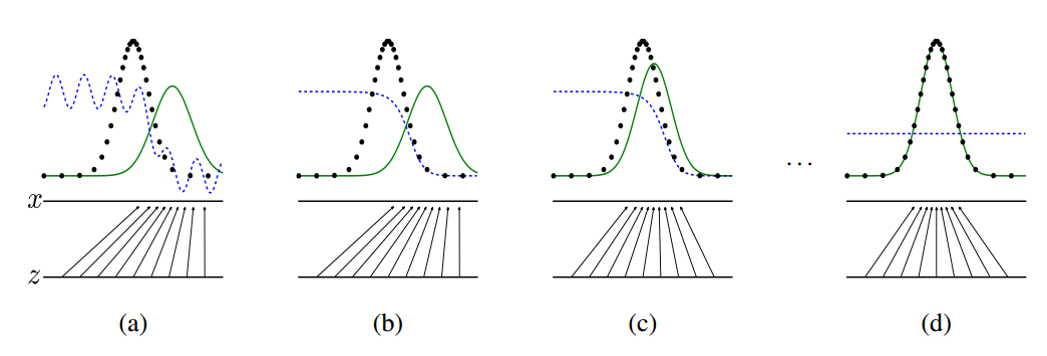这张图表明的是GAN的生成网络如何一步步从均匀分布学习到正太分布的。原始数据x服从正太分布，这个过程你也没告诉生成网络说你得用正太分布来学习，但是生成网络学习到了。假设你改一下x的分布，不管什么分布，生成网络可能也能学到。这就是GAN可以自动学习真实数据的分布的强大之处。
还有人说GAN强大之处在于可以自动的定义潜在损失函数。 什么意思呢，这应该说的是判别网络可以自动学习到一个好的判别方法，其实就是等效的理解为可以学习到好的损失函数，来比较好或者不好的判别出来结果。虽然大的loss函数还是我们人为定义的，基本上对于多数GAN也都这么定义就可以了，但是判别网络潜在学习到的损失函数隐藏在网络之中，不同的问题这个函数就不一样，所以说可以自动学习这个潜在的损失函数。
开始做小实验
本节主要实验一下如何通过随机数组生成mnist图像。mnist手写体数据库应该都熟悉的。这里简单的使用matlab来实现，方便看到整个实现过程。这里用到了一个工具箱  DeepLearnToolbox,关于该工具箱的一些其他使用说明
网络结构很简单，就定义成下面这样子：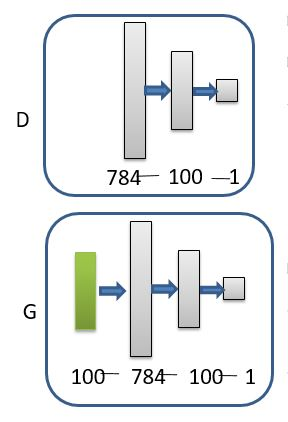将上述工具箱添加到路径，然后运行下面代码：
clc
clear
%% 构造真实训练样本 60000个样本 1*784维（28*28展开）

train_x = double(train_x(1:60000,:)) / 255;
% 真实样本认为为标签 [1 0]； 生成样本为[0 1];
train_y = double(ones(size(train_x,1),1));
% normalize
train_x = mapminmax(train_x, 0, 1);

rand('state',0)
%% 构造模拟训练样本 60000个样本 1*100维
test_x = normrnd(0,1,[60000,100]); % 0-255的整数
test_x = mapminmax(test_x, 0, 1);

test_y = double(zeros(size(test_x,1),1));
test_y_rel = double(ones(size(test_x,1),1));

%%
nn_G_t = nnsetup([100 784]);
nn_G_t.activation_function = 'sigm';
nn_G_t.output = 'sigm';

nn_D = nnsetup([784 100 1]);
nn_D.weightPenaltyL2 = 1e-4;  %  L2 weight decay
nn_D.dropoutFraction = 0.5;   %  Dropout fraction
nn_D.learningRate = 0.01;                %  Sigm require a lower learning rate
nn_D.activation_function = 'sigm';
nn_D.output = 'sigm';
% nn_D.weightPenaltyL2 = 1e-4;  %  L2 weight decay

nn_G = nnsetup([100 784 100 1]);
nn_G.weightPenaltyL2 = 1e-4;  %  L2 weight decay
nn_G.dropoutFraction = 0.5;   %  Dropout fraction
nn_G.learningRate = 0.01;                %  Sigm require a lower learning rate
nn_G.activation_function = 'sigm';
nn_G.output = 'sigm';
% nn_G.weightPenaltyL2 = 1e-4;  %  L2 weight decay

opts.numepochs =  1;        %  Number of full sweeps through data
opts.batchsize = 100;       %  Take a mean gradient step over this many samples
%%
num = 1000;
tic
for each = 1:1500
%----------计算G的输出：假样本-------------------
for i = 1:length(nn_G_t.W)   %共享网络参数
nn_G_t.W{i} = nn_G.W{i};
end
G_output = nn_G_out(nn_G_t, test_x);
%-----------训练D------------------------------
index = randperm(60000);
train_data_D = [train_x(index(1:num),:);G_output(index(1:num),:)];
train_y_D = [train_y(index(1:num),:);test_y(index(1:num),:)];
nn_D = nntrain(nn_D, train_data_D, train_y_D, opts);%训练D
%-----------训练G-------------------------------
for i = 1:length(nn_D.W)  %共享训练的D的网络参数
nn_G.W{length(nn_G.W)-i+1} = nn_D.W{length(nn_D.W)-i+1};
end
%训练G：此时假样本标签为1，认为是真样本
nn_G = nntrain(nn_G, test_x(index(1:num),:), test_y_rel(index(1:num),:), opts);
end
toc
for i = 1:length(nn_G_t.W)
nn_G_t.W{i} = nn_G.W{i};
end
fin_output = nn_G_out(nn_G_t, test_x);

函数nn_G_out为：
function output = nn_G_out(nn, x)
nn.testing = 1;
nn = nnff(nn, x, zeros(size(x,1), nn.size(end)));
nn.testing = 0;
output = nn.a{end};
end

看一下这个及其简单的函数，其实最值得注意的就是中间那个交替训练的过程，这里我分了三步列出来：
重新计算假样本（假样本每次是需要更新的，产生越来越像的样本）训练D网络，一个二分类的神经网络；训练G网络，一个串联起来的长网络，也是一个二分类的神经网络（不过只有假样本来训练），同时D部分参数在下一次的时候不能变了。
就这样调一调参数，最终输出在fin_output里面，多运行几次显示不同运行次数下的结果：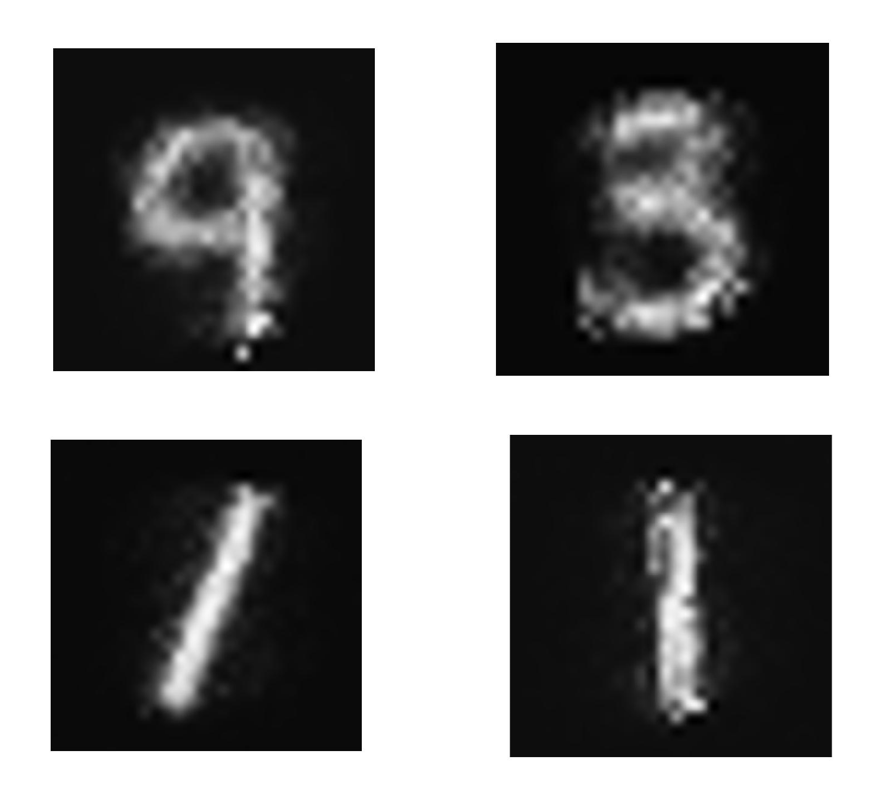可以看到的是结果还是有点像模像样的。
实验总结
运行上述简单的网络我发现几个问题：
网络存在着不收敛问题；网络不稳定；网络难训练；读过原论文其实作者也提到过这些问题，包括GAN刚出来的时候，很多人也在致力于解决这些问题，当你实验自己碰到的时候，还是很有意思的。那么这些问题怎么体现的呢，举个例子，可能某一次你会发现训练的误差很小，在下一代训练时，马上又出现极限性的上升的很厉害，过几代又发现训练误差很小，震荡太严重。其次网络需要调才能出像样的结果。交替迭代次数的不同结果也不一样。比如每一代训练中，D网络训练2回，G网络训练一回，结果就不一样。这是简单的无条件GAN，所以每一代训练完后，只能出现一个结果，那就是0-9中的某一个数。要想在一代训练中出现好几种结果，就需要使用到条件GAN了。
最后
现在的GAN已经到了五花八门的时候了，各种GAN应用也很多，理解底层原理再慢慢往上层扩展。GAN还是一个很厉害的东西，它使得现有问题从有监督学习慢慢过渡到无监督学习，而无监督学习才是自然界中普遍存在的，因为很多时候没有办法拿到监督信息的。要不Yann Lecun赞叹GAN是机器学习近十年来最有意思的想法。
福利
该节部分出了个视频版的讲解，详情请点击：http://www.mooc.ai/open/course/301  欢迎关注【微信公众号：AInewworld】了解更多。
展开全文深度学习
• Wasserstein GAN论文一篇，人工智能深度学习爱好者取阅。无监督学习
• GAN GAN 有两个网络，一个是 generator，一个是 discriminator，通过两个网络互相对抗来达到最好的生成效果。 公式： 先固定 G，来求解最优的 D 对于一个给定的 x，得到最优的 D 如上图，范围在 (0,1) 内，......